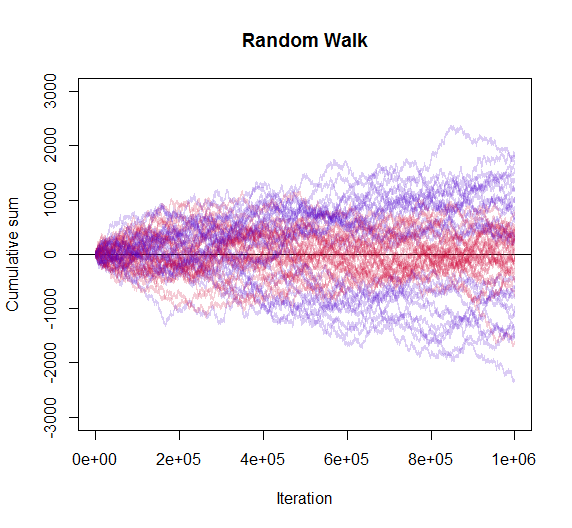### PROBABILITY TO RETURN TO THE ORIGIN IN A SYMMETRIC RANDOM WALK:To return to the origin, an even number of steps are needed. The probability of being at the origin at a particular step $$2n$$ is:

$\color{turquoise}{\large p_{2n}\overset{\Delta}{=}P(X_{2n}=0, n=0,1,2,\cdots)= {2n \choose n}\,\Big(pq\Big)^n} \tag 1$

$$p$$ and $$q$$ are the Berouilli probabilities to turn right or left. They are equal in a symmetric random walk.

The first return happening at step $$2n$$ will have a probability:

$\color{turquoise}{\large f_{2n}\overset{\Delta}{=}P(x_{2n}=0 \cap X_{2k}\neq 0 \, \forall k<n)} \tag 2$

The initial state is $$f_0=0$$ and $$p_0=1$$.

There are $$\large p_{2n}\,2^{2n}$$ paths of length $$2n$$ between point $$(0,0)$$ and $$(2n,0)$$. In the case of a symmetric random walk all paths have the same probability: $$\large (\frac{1}{2})^{2n}$$. Therefore:

$\large p_{2n}={2n \choose n}\,2^{-2n}.$

We can partition these paths depending on the time of the first pass. If the first return is on step $$2k$$, there is a segment from $$(0,0)$$ to $$(2k,0)$$ with no returns, and from $$(2k,0)$$ to $$(2n,0)$$ without restrictions.

The number of paths with first pass at $$2k$$ are:

$\large f_{2k}\,2^{2k} \times p_{2n-2k}\,2^{2n-2k}= f_{2k}\,p_{2n-2k}\,2^{2n}$

Summing over $$k$$:

$\large p_{2n}\, 2^{2n}=f_0\,p_{2n}\,2^{2n}+f_2\,p_{2n-2}\,2^{2n}+\cdots+f_{2n}p_0\, 2^{2n}$

Dividing both sides by $$2^{2n}$$:

$\color{blue}{\large p_{2n}=f_0\,p_{2n}+f_2\,p_{2n-2}+\cdots+f_{2n}p_0}\tag 2$

This relates the probability of return to origin at time $$2n$$ to first and other returns.

Based on this theorem we can set up the following convolution:

$\large p_{n}=\displaystyle\sum_{k=1}^n\,f_k\times p_{n-k}$

and set up the following generating functions from the power series (credit to this post):

\begin{eqnarray*} P(x)&=&\sum_{n=0}^\infty p_n x^n\$5pt] &=&1+\sum_{n=1}^\infty p_n x^n\\[5pt] &=&1+\sum_{n=1}^\infty \sum_{k=1}^n f_k p_{n-k} x^n\\[5pt] &=&1+\sum_{k=1}^\infty \sum_{n=k}^\infty f_k p_{n-k} x^n\\[5pt] &=&1+\sum_{k=1}^\infty f_k x^k \sum_{n=k}^\infty p_{n-k} x^{n-k}\\[5pt] &=&1+\sum_{k=0}^\infty f_k x^k \sum_{j=0}^\infty p_{j} x^{j}\\[5pt] &=&\Large \color{orange}{1+F(x) P(x)}\tag 3 \end{eqnarray*} Now, \[\large \color{blue}{\displaystyle\sum_{n=0}^\infty\,{2n\choose n}\,x^n=\frac{1}{\sqrt{1-4x}}=(1-4x)^{-1/2}}\tag 4$

PROOF (thanks this post)

To see this we get the Taylor series, $$\large f(x)=\sum_{n=0}^{\infty}\frac{f^{(n)}(x_0)}{n!}(x-x_0)^n$$, of this expression evaluated at $$0$$:

$$f(x)=(1-4x)^{-1/2}$$; evaluated at $$f(0)=1.$$

$$f^1(x)=\frac{2}{(1-4x)^{3/2}}$$; evaluated at $$f^1(0)=2$$; coefficient $$2/1!$$.

$$f^2(x)=\frac{12}{(1-4x)^{5/2}}$$; evaluated at $$f^2(0)=12$$; coefficient $$12/2!=6$$.

$$f^3(x)=\frac{120}{(1-4x)^{7/2}}$$; evaluated at $$f^3(0)=120$$; coefficient $$120/3!=20$$

$$f^4(x)=\frac{1680}{(1-4x)^{9/2}}$$; evaluated at $$f^4(0)=1680$$; coefficient $$1680/4!=70$$.

The Taylor series is:

$$1+2 x+6 x^2+20 x^3+70 x^4+O\left(x^5\right)$$

and the coefficients are $$\Large \frac{2n!}{n!n!}={2n\choose n}.$$

$$\square$$

Consequently, from equation $$(1)$$:

$\color{purple}{\large P(x)= \displaystyle\sum_{n=0}^\infty=\,p_n\,x^n=\binom{2n} {n}\,(pqx)^n=(1-4pqx)^{-1/2}}\tag 5$

and going back to equation 3:

$F(x)=1-\frac{1}{P(x)}$

and

$\color{purple}{\large F(x)=1 - (1-4pqx)^{1/2}}\tag 6$

Next:

$\large \color{red}{\sqrt{1-x}=\displaystyle\sum_{n=0}^\infty\frac{-1}{2n-1}\,\binom{2n}{n}\,\frac{x^n}{4^n}}\tag 7$

PROOF (thanks to this post):

Let $$f(x)=(1-x)^{1/2}$$. Differentiating:

\begin{align} f^{(1)}(x)&=(-1)\left(\frac12\right) (1-x)^{-1/2}\\\\ f^{(2)}(x)&=(-1)^2\left(\frac12\right) \left(-\frac12\right) (1-x)^{-3/2} \\\\ f^{(3)}(x)&=(-1)^3\left(\frac12\right) \left(-\frac12\right) \left(-\frac32\right) (1-x)^{-5/2}\\\\ f^{(4)}(x)&=(-1)^4\left(\frac12\right) \left(-\frac12\right) \left(-\frac32\right)\left(-\frac52\right) (1-x)^{-7/2}\\\\ \vdots\\\\ f^{(n)}(x)&=-\frac{(2n-3)!!}{2^n}(1-x)^{-(2n-1)/2} \\\\ \end{align}

Therefore $$f(x)$$ has the series expansion:

$$\large f(x)=1-\sum_{n=1}^\infty \frac{(2n-3)!!}{2^n\,n!}\,x^n \tag {*}$$

with

\begin{align} (2n-3)!!&=(2n-3)(2n-5)(2n-7)\cdots(5)(3)(1)\\\\ &=\frac{(2n-3)!}{(2n-4)(2n-6)(2n-8)\cdots (6)(4)(2)}\\\\ &=\frac{(2n-3)!}{2^{n-2}(n-2)!}\\\\ &=\frac{(2n)!}{(2n-1)2^n\,n!}\\\\ &=\frac{n!}{(2n-1)2^n}\binom{2n}{n} \tag {**} \end{align}

Substituting (**) into (*):

\begin{align} f(x)&=1-\sum_{n=1}^\infty \frac{1}{4^n(2n-1)}\binom{2n}{n}\,x^n\\\\ &=-\sum_{n=1}^\infty \frac{1}{4^n(2n-1)}\binom{2n}{n}\,x^n \end{align}

$$\square$$

Now substituting into $$(6)$$ into $$(5)$$:

$\large F(x)=\displaystyle \sum_{n=0}^\infty f_n x^n= \sum_{n=1}^\infty \frac{1}{2n-1}{2m\choose n}(pq)^n\,x^n\tag 8$

Therefore,

$\color{LimeGreen}{\large f_n=\frac{1}{2n-1} \binom{2n}{n} (pq)^n} \tag 9$

A probability generating function for a random variable $$X$$ taking values in $$\{0,\infty\}$$ will converge at $$x=1$$. So at $$x=1$$ the sum of all the $$f_n$$ is simply the probability that the random walk ever returning to the origin (by equation $$(5)$$):

$\color{red}{\large F(1)=\displaystyle \sum_{n=1}^\infty f_n= 1 - \sqrt{1-4pq}=1-}\color{red} {|p-q|}\tag {10}$

Here I resort to this post:

$$(p-q)^2=p^2-2pq+q^2=p(1-q)-2pq+q(1-p)=p+q-4pq=1-4pq$$

With probability $$|p-q|$$ the walk will never return to the origin. If $$p=q$$, the probability of not returning is zero.